Tamilnadu State Board New Syllabus Samacheer Kalvi 11th Physics Guide Pdf Chapter 8 Heat and Thermodynamics Text Book Back Questions and Answers, Notes.

## Tamilnadu Samacheer Kalvi 11th Physics Solutions Chapter 8 Heat and Thermodynamics

### 11th Physics Guide Heat and Thermodynamics Book Back Questions and AnswersPart – I:
I. Multiple choice questions:

Question 1.
In hot summer after a bath, the body’s:
(a) internal energy decreases
(b) internal energy increases
(c) heat decreases
(d) no change in internal energy and heat
(a) internal energy decreases

Question 2.
The graph between volume and temperature in Charle’s law is:
(a) an ellipse
(b) a circle
(c) a straight line
(d) a parabola
(c) a straight lineQuestion 3.
When a cycle tyre suddenly bursts, the air inside the tyre expands. This process is:
(a) isothermal
(c) isobaric
(d) isochoric

Question 4.
An ideal gas passes from one equilibrium state (P1, V1, T1, N) to another equilibrium state (2P1, 3V1, T2, N). Then:
(a) T1 = T2
(b) T1 = $$\frac{\mathrm{T}_{2}}{6}$$
(c) T1 = 6T2
(d) T1 = 3T2
(b) T1 = $$\frac{\mathrm{T}_{2}}{6}$$

Question 5.
When a uniform rod is heated, which of the following quantity of the rod will increase:
(a) mass
(b) weight
(c) centre of mass
(d) moment of inertia
(d) moment of inertia

Hint:
M.I. = MK²
During heating K would be increased. Hence moment of inertia will increase.Question 6.
When food is cooked in a vessel by keeping the lid closed, after some time the steam pushes the lid outward. By considering the steam as a thermodynamic system, then in the cooking process:
(a) Q > 0, W > 0
(b) Q < 0, W > 0
(c) Q > 0, W < 0
(d) Q < 0, W < 0
(a) Q > 0, W > 0

Hint:
Q > 0; W > 0
∆Q = ∆U1 + ∆W.
∴ ∆Q ∝ ∆W
When ∆Q > 0
∆W > 0.

Question 7.
When you exercise in the morning, by considering your body as a thermodynamic system, which of the following is true?
(a) ∆U > 0, W > 0
(b) ∆U < 0, W > 0
(c) ∆U< 0, W < 0
(d) ∆U = 0, W > 0
(b) ∆U < 0, W > 0

Hint:
∆Q = ∆U + ∆W
When exercise is performed, internal energy is utilised (∆U < 0). So it will decrease.
But as internal energy is utilised, the exercise (∆W) will be more. So work will be more.
∴ ∆W > 0
∆U < 0 W > 0Question 8.
A hot cup of coffee is kept on the table. After some time it attains a thermal equilibrium with the surroundings. By considering the air molecules in the room as a thermodynamic system, which of the following is true?
(a) ∆U > 0, Q = 0
(b) ∆U > 0, W < 0
(c) ∆U > 0, Q > 0
(d) ∆U = 0, Q > 0
(c) ∆U > 0, Q > 0

Hint: During the thermal equilibrium with surrounding as heat energy (Q) is increased, internal energy will be increased.
∴ ∆U > 0 Q > 0

Question 9.
An ideal gas is taken from (Pi, Vi) to (Pf, Vf) in three different ways. Identify the process in which the work done on the gas the most.(a) Process A
(b) Process B
(c) Process C
(d) Equal work is done in Process A, B & C
(b) Process B

Hint:
When work is done on the gas, compression takes place. Final pressure Pf will be increased and remains constant. It is shown by process B.

Question 10.
The V-T diagram of an ideal gas which goes through a reversible cycle A → B → is shown below. (Processes D → A and B → C are adiabatic)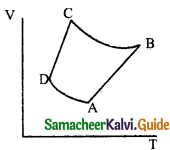The corresponding PV diagram for the process is (all figures are schematic)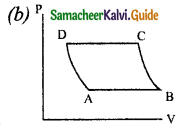Question 11.
A distant star emits radiation with maximum intensity at 350 nm. The temperature of the star is:
(a) 8280 K
(b) 5000 K
(c) 7260 K
(d) 9044 K
(a) 8280 K

Hint:Question 12.
Identify the state variables given here.
(a) Q, T, W
(b) P,T,U
(c) Q, W
(d) P,T,Q
(b) P,T,U

Question 13.
In an isochoric process, we have:
(a) W = 0
(b) Q = 0
(c) ∆U = 0
(d) ∆T = 0
(a) W = 0

Hint:
Work done in an isochoric process is zero.

Question 14.
The efficiency of a heat engine working between the freezing point and boiling point of water is: (NEET2018)
(a) 6.25%
(b) 20%
(c) 26.8%
(d) 12.5%
(b) 20%

Hint:
T2 = 0° C = 0 + 273 = 273 K
T1 = 100° C = 100 + 273 = 373 KQuestion 15.
An ideal refrigerator has a freezer at a temperature of -12°C. The coefficient of performance of the engine is 5. The temperature of the air (to which the heat ejected) is:
(a) 50°C
(b) 45.2°C
(c) 40.2°C
(d) 37.5°C
(c) 40.2°C

Hint: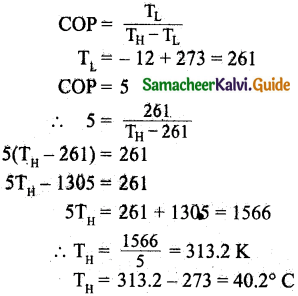Question 1.
‘An object contains more heat’- is it a right statement? If not why?
When heated, an object receives heat from the agency. Now object has more internal energy than before. Heat is the energy in transit and which flows from an object at a higher temperature to an object at lower temperature. Heat is not a quantity. So the statement I would prefer “an object contains more thermal energy”.

Question 2.
Obtain an ideal gas law from Boyle’s and Charles’law.
According to Boyle’s law, Pressure ∝ $$\frac { 1 }{ 2 }$$
i.e., P ∝ $$\frac { 1 }{ V }$$
According to Charle’s law,
Volume ∝ Temperature, i.e., V ∝ T
By combining these two laws, we get
PV= CT … (1)
Where C is a positive constant
But C = k x Number of particles (N)
C = kN
Where k – Boltzmann’s constant.
∴ Equation (1) becomes
PV = NkTQuestion 3.
Define one mole.
One mole of any substance is the amount of that substance which contains Avogadro number (NA) of particles (such as atoms or molecules).

Question 4.
Define specific heat capacity and give its unit.
Specific heat capacity of a substance is defined as the amount of heat energy required to raise the temperature of 1 kg of a substance by 1 Kelvin or 1 °C.
The SI unit for specific heat capacity is J kg-1 K-1.

Question 5.
Define molar specific heat capacity.
Heat energy required to increase the temperature of one mole of substance by IK or 1°C.

Question 6.
What is a thermal expansion?
Thermal expansion is the tendency of matter to change in shape, area, and volume due to a change in temperature.

Question 7.
Give the expressions for linear, area and volume thermal expansions.
(i) Linear expansion:
$$\frac { ∆L }{ L }$$ = α ∆T ⇒ αL = $$\frac { ∆L }{ L∆T }$$

(ii) Area expansion:
$$\frac { ∆A }{ A }$$ = 2α ∆T ⇒ αA = $$\frac { ∆A }{ A∆T }$$

(iii) Volume expansion:
$$\frac { ∆V }{ V }$$ = 3α ∆T ⇒ αv = $$\frac { ∆V }{ V∆T }$$

Question 8.
Define latent heat capacity. Give its unit.
Latent heat capacity of a substance is defined as the amount of heat energy required to change the state of a unit mass of the material. The SI unit for latent heat capacity is J kg-1.Question 9.
State Stefan-Boltzmann law.
The total amount of heat energy radiated per second per unit area of a black body is directly proportional to the fourth power of its absolute temperature.

Question 10.
What is Wien’s law?
The wavelength of maximum intensity of emission of a black body radiation is inversely proportional to the absolute temperature of the black body.

Question 11.
Define thermal conductivity. Give its unit.
The quantity of heat transferred through a unit length of a material in a direction normal to Unit surface area due to a unit temperature difference under steady-state conditions is known as the thermal conductivity of a material.

Question 12.
What is a black body?
A black body is one which neither reflects nor transmits but absorbs whole of the radiation incident on it.

Question 13.
What is a thermodynamic system? Give examples.
Thermodynamic system: A thermodynamic system is a finite part of the universe. It is a collection of a large number of particles (atoms and molecules) specified by certain parameters called pressure (P), Volume (V), and Temperature (T). The remaining part of the universe is called the surrounding. Both are separated by a boundary.
Examples: A thermodynamic system can be liquid, solid, gas, and radiation.

Question 14.
What are the different types of thermodynamic systems?
(i) Open system can exchange both matter and energy with the environment.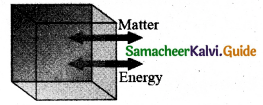(ii) Closed system exchange energy but not matter with the environment.(iii) Isolated system can exchange neither energy nor matter with the environment.Question 15.
What is meant by ‘thermal equilibrium’?
Two systems are said to be in thermal equilibrium with each other if they are at the same temperature, which will not change with time.

Question 16.
What is mean by state variable? Give example.
The quantities that are used to describe the equilibrium states of a Thermodynamic system. .
Example: Pressure, Volume, Temperature.

Question 17.
What are intensive and extensive variables? Give examples.
Extensive variable depends on the size or mass of the system.
Example: Volume, total mass, entropy, internal energy, heat capacity etc.
Intensive variables do not depend on the size or mass of the system.
Example: Temperature, pressure, specific heat capacity, density etc.

Question 18.
What is an equation of state? Give an example.
The equation that relates the state variables in a specific manner is called equation of state.
Examples:
(i) Gas equation
PV = NkT

(ii) Vander Waal’s equation, .
(p +$$\frac{a}{v^{2}}$$)(v – b) = RTQuestion 19.
State Zeroth law of thermodynamics,
The zeroth law of thermodynamics states that if two systems, A and B, are in thermal equilibrium with a third system C, then A and B are in thermal equilibrium with each other.

Question 20.
Define the internal energy of the system.
The sum of kinetic and potential energies of all the molecules of the system with respect to the centre of mass of the system.

Question 21.
Are internal energy and heat energy the same? Explain.
Internal energy and thermal energy do not mean the same thing, but they are related. Internal energy is the energy stored in a body. It increases when the temperature of the body rises, or when the body changes from solid to liquid or from liquid to gas.
“Heat is the energy transferred from one body to another as a result of a temperature difference.”

Question 22.
Define one calorie.
To raise 1 g of an object by 1°C , 4.186 J of energy is required. In earlier days the heat energy was measured in calorie.
1 cal = 4.186J

Question 23.
Did joule converted mechanical energy to heat energy? Explain.
In Joule’s experiment, when the masses fall, the paddle wheel turns. The frictional force between paddle wheel and water causes a rise in temperature of water. It is due to the work done by the masses. This infers that gravitational potential energy is transformed into internal energy of water. Thus Joule converted mechanical energy into heat energy.Question 24.
State the first law of thermodynamics.
‘Change in internal energy (AU) of the system is equal to heat supplied to the system (Q) minus the work done by the system (W) on the surroundings’.

Question 25.
Can we measure the temperature of the object by touching it?
No, we can’t measure the temperature of the object touching it. Because the temperature is the degree of hotness or coolness of a body. Only we can sense the hotness or coolness of the object.

Question 26.
Give the sign convention for Q and W.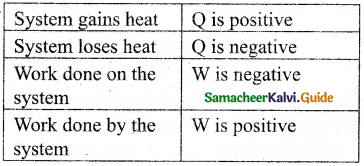Question 27.
Define the quasi-static process.
A quasi-static process is an infinitely slow process in which the system changes its variables (P, V, T) so slowly such that it remains in thermal, mechanical, and chemical equilibrium with its surroundings throughout.

Question 28.
Give the expression for work done by the gas.
In general, the work done by the gas by increasing the volume from Vi to Vf is given by
W = $$\int_{V_{i}}^{V_{f}} \mathrm{P} d \mathrm{~V}$$

Question 29.
What is PV diagram?
PV diagram is a graph between pressure P and volume V of the system. The P-V diagram is used to calculate the amount of work done by the gas during expansion or on the gas during compression.

Question 30.
Explain why the specific heat capacity at constant pressure is greater than the specific heat capacity at constant volume.
When increasing the temperature of a gas at constant pressure, gas expands and consume some heat to do work. It is not happened, while increasing the temperature of a gas at constant volume. Hence less heat energy is required to increase the temperature of the gas at constant volume. Hence CP is always greater than Cv.

Question 31.
State the equation of state for an isothermal process.
The equation of state for isothermal process is given by,
PV = constant

Question 32.
Give an expression for work done in an isothermal process.
W = μRTln $$\left(\frac{\mathrm{V}_{f}}{\mathrm{~V}_{i}}\right)$$Question 33.
Express the change in internal energy in terms of molar specific heat capacity.
When the gas is heated at the constant volume the temperature increases by dT. As no work is done by the gas, the heat that flows into the system will increase only the internal energy. Let the change in internal energy be dU. dU = µωdT

Question 34.
Apply first law for (i) an isothermal (ii) adiabatic (Hi) isobaric processes.
(i) For an isothermal process temperature is maintained as constant. Hence,
∆V= 0
∴ W = P∆V = 0
According to first law of thermodynamics
∆U = Q – W
= Q – 0
= Q
∴ ∆U = Q

(ii) For adiabatic process, Q = 0
By applying first law of thermodynamics
∆U = Q – W
∆U = |- W| = W
∆U = W = $$\frac{\mu R}{\gamma-1}$$ = (Ti – Tf)

(iii) (a) For isobaric expansion Q > O
According to first law of thermodynamics
∆U = – Q – W
∆U = Q – P∆V

(b) For isobaric compression Q < O.
According to first law of thermodynamics
∆U = – Q – W
= – Q – P∆V

Question 35.
Give the equation of state for an adiabatic process.
The equation of state for an adiabatic proc ess is given by,
PVγ = Constant

Question 36.
State an equation state for an isochoric process.
The equation of state for an isochoric process is given by,
P = ($$\frac { μR }{ V }$$)T

Question 37.
if the piston of a container is pushed fast inward. Will the ideal gas equation be valid in the intermediate stage? ff not, why?
When the piston is compressed so quickly that there is no time to exchange heat to the surrounding, the temperature of the gas increases rapidly. In this intermediate stage the ideal gas equation be not valid. Because this equation can be relates the pressure, volume and temperature of thermodynamic system at equilibrium.Question 38.
Draw the PV diagram for,
(a) isothermal process
(c) lsobaric process
(d) lsochoric process
(a) isothermal process: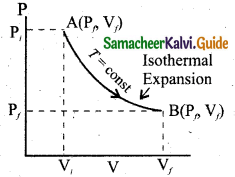(c) lsobaric process:(d) lsochoric process: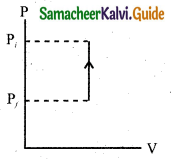Question 39.
What is a cyclic process?
It is a process in which the thermodynamic system returns to its initial state after undergoing a series of changes.

Question 40.
What is meant by a reversible and irreversible processes?
Reversible processes: A thermodynamic process can be considered reversible only if it possible to retrace the path in the opposite direction in such a way that the system and surroundings pass through the same states as in the initial, direct process.
Irreversible processes: All natural processes are irreversible. Irreversible process cannot be plotted in a PV diagram, because these processes cannot have unique values of pressure, temperature at every stage of the process.

Question 41.
State Clausius form of the second law of thermodynamics.
“Heat energy always flows from hotter object to colder object spontaneously”. This is known as the Clausius form of second law of thermodynamics.

Question 42.
State Kelvin-Planck statement of second law of thermodynamics.
It is impossible to construct a heat engine that operates in a cycle, whose sole effect is to convert the heat completely into work.

Question 43.
Define heat engine.
Heat engine is a device which takes heat as input and converts this heat in to work by undergoing a cyclic process.

Question 44.
What are processes involves in a Carnot engine?

1. Isothermal expansion
3. Isothermal compression

Question 45.
Can the given heat energy be completely converted to work in a cyclic process? If not, when can the heat can completely converted to work?
No.
According to second law it can’t be completely converted. It is not possible to convert heat completely converted into work, in a cyclic process. But, in Non-cyclic process, heat can be completely converted into work.Question 46.
State the second law of thermodynamics in terms of entropy.
“For all the processes that occur in nature (irreversible process), the entropy always increases. For reversible process entropy will not change”. Entropy determines the direction in which natural processes should occur.

Question 47.
Why does heat flow from a hot object to a cold object?
Because entropy increases when heat flows from hot object to cold object. If heat were to flow from a cold to a hot object, entropy will be decreased. So second law thermodynamics is violated.

Question 48.
Define the coefficient of performance.
The ratio of heat extracted from the cold body (sink) to the external work done by the compressor W.
COP = ß = $$\frac{\mathrm{Q}_{\mathrm{L}}}{\mathrm{W}}$$.

Question 1.
Explain the meaning of heat and work with suitable examples.
The spontaneous flow of energy from the object at higher temperature to the one at lower temperature. When they are contact. This energy is called heat. This process of energy transfer from higher temperature object to lower temperature object is called heating.

Example: ‘A hot cup of coffee has more heat’. This statement is correct or wrong?

When heated, a cup of coffee receives heat from the stove. Once the coffee is taken from the stove, the cup of coffee has more internal energy than before. ‘Heat’ is the energy in transit and that flows from a body at higher temperature to an other body at lower temperature. Heat is not a quantity. So the statement ‘A hot cup of coffee has more heat’ is wrong, instead ‘coffee is hot’ will be appropriate.

Meaning of Work: If a body undergoes a displacement then only work is said to be done by the body. Work is also the transfer of energy by other means such as moving a piston of a cylinder containing gas.

Example: When you rub your hands against each other the temperature of the hand is increased. You have done some work on your hands by rubbing. The temperature of the hands increases due to this work. Now when you place your hands on the chin, the temperature of the chin increases.

This is because the hands are at higher temperature than the chin. In the above example, the temperature of hands is increased due to work and temperature of the chin is increased due to heat transfer from the hands to the chin.

The temperature in the system will increase and sometimes may not by doing work on the system. Like heat, work is also not a quantity and through the work energy is transferred to the system.Question 2.
Discuss the ideal gas laws.
(i) Boyles law: When the gas is kept at constant temperature, the pressure of the gas is inversely proportional to the volume.
P ∝ $$\frac { 1 }{ v }$$

(ii) Charles law: When the gas is kept at constant pressure, the volume of the gas is directly proportional to absolute temperature. V ∝ T

(iii) By combining these two equations we have PV= CT … (1)

(iv) Let the constant C as k times the number of particles N. Here k is the Boltzmann constant and it is found to be a universal constant. So the ideal gas law can be stated as follows,
PV = NkT … (2)
If the gas consists of p mole of particles then total number of particles is
N = μNA … (3)
Substituting the value of N in the equation (2) we get
PV = μNAkT
Here NAk = R
So, the ideal gas law can be written for μ mole of gas is
PV= pRT
It is called equation of state for an ideal gas.

Question 3.
Explain in detail the thermal expansion.
Thermal expansion is the tendency of matter to change in shape, area, and volume due to a change in temperature. When a solid is heated, its atoms vibrate with higher amplitude about their fixed points. At that time, relative change in the size of solids is small.

Liquids expand more than solids because, they have less intermolecular forces than solids. In the case of gas molecules, the intermolecular forces are almost negligible and so they expand much more than solids.

Linear Expansion: In solids, for a small change in temperature ∆T, the fractional change in length $$\frac { ∆L }{ L }$$ is directly proportional to ∆T.
$$\frac { ∆L }{ L }$$ = αL∆T
Therefore, αL = $$\frac { ∆L }{ L∆T }$$

Area Expansion: For an infinitesimal change in temperature ∆T the fractional change in area of a substance is directly proportional to ∆T and it can be written as
$$\frac { ∆A }{ A }$$ = αA∆T
Therefore, αA = $$\frac { ∆A }{ A∆T }$$
Volume Expansion: For a very small change in temperature ∆T the fractional change in volume $$\frac { ∆V }{ V }$$ of a substance is directly proportional to AT.
$$\frac { ∆V }{ V }$$ = αV∆T
Therefore, αV = $$\frac { ∆L }{ V∆T }$$Question 4.
Describe the anomalous expansion of water. How is it helpful in our lives?
On heating liquids expand and contract on cooling at moderate temperatures. But water exhibits an anomalous behavior. It contracts on heating between 0°C and 4°C. The volume of the given amount of water decreases as . it is cooled from room temperature, until it reach 4°C . Below 4°C the volume increases and so the density decreases. It means that the water has a maximum density at 4°C. This behaviour of water is called anomalous expansion of water.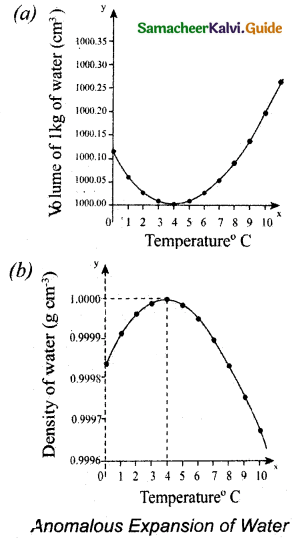During winter in cold countries, the surface of the lakes would be at lower temperature than the bottom. Since the solid water (ice) has lower density than its liquid form,’below 4°C, the frozen water will be on the top surface above the liquid water (ice floats). It is due to the anomalous expansion of water. The water in lakes and ponds freeze only at the top. So, the species living in the lakes will be safe at the bottom.

Question 5.
Explain Calorimetry and derive an expression for final temperature when two thermodynamic systems are mixed.
Calorimetry means the measurement of the amount of heat released or absorbed by thermodynamic system during the heating process. When a body at higher temperature is brought in contact with another body at lower temperature, due to transformation of heat. The heat lost by the hot body is equal to the heat gained by the cold body. No heat is allowed to escape to the surroundings. It can be mathematically expressed as
Qgain = – Qlost
Qgain + Qlost = 0
Heat gained or lost can be measured with a calorimeter.
When a sample is heated at high temperature (T1) and immersed into water at room temperature (T2) in the calorimeter. After some time both sample and water reach a final equilibrium temperature Tf. Since the calorimeter is insulated, heat given by the hot sample is equal to heat gained by the water.
Qgain = – Qlost
As per the sign convention, the heat energy lost is denoted by negative sign and heat gained is denoted as positive.
From the definition of specific heat capacity,
Qgain= m2s2(Tf – T2)
Qlost = m1s1(Tf – T1)
Here s1 and s2 specific heat capacity of hot sample and water respectively.
So we can write,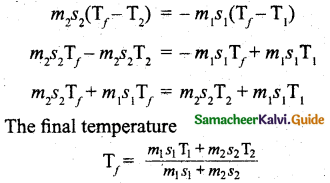Question 6.
Discuss various modes of heat transfer.
There are three modes of heat transfer: Conduction, Convection and Radiation.

Conduction: Conduction is the process of direct transfer of heat through matter due to temperature difference. If two objects are placed in direct contact with one another, then heat will be transferred from the hotter object to the colder one.

Convection: Convection is the process in which heat energy transfer is by actual movement of molecules in fluids such as liquids and gases. In convection, molecules move freely from one place to another.
Example: Boiling of water in a pot.

Radiation: Radiation is a form of energy transfer from one body to another by electromagnetic waves.
Example: Solar energy from the Sun.Question 7.
Explain in detail Newton’s law of cooling.
Newton’s law of cooling states that the rate of loss of heat of a body is directly proportional to the difference in the temperature between that body and its surroundings.
$$\frac { dQ }{ dt }$$ ∝ – (T – Ts) … (1)
The negative sign implies that the quantity of heat lost by liquid goes on decreasing with time. Where,
T = Temperature of the object
T = Temperature of the surrounding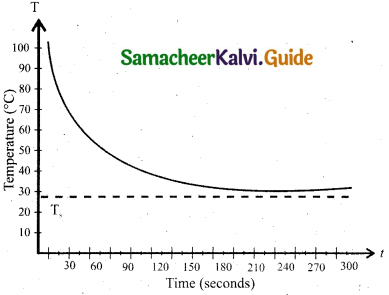From the above graph, it is clear that the rate of cooling is high initially and decreases with falling temperature.

Consider a body of mass m and specific heat capacity s at temperature T. Let T be the temperature of the surroundings. If the temperature falls by a small amount dl in time dt, then the amount of heat lost is,
dQ = msdT
Dividing both sides of equation (2) by dt
$$\frac { dQ }{ dt }$$ ∝ $$\frac { msdT }{ dt }$$ … (3)
From Newton’s law of cooling
$$\frac { dQ }{ dt }$$ ∝ (T – Ts)
$$\frac { dQ }{ dt }$$ ∝ (T – Ts) … (4)
Where a is some positive constant.
From equation (3) and (4)
– a(T – Ts) = ms$$\frac { dT }{ dt }$$
$$\frac{d \mathrm{~T}}{\mathrm{~T}-\mathrm{T}_{s}}$$ = – $$\frac { a }{ ms }$$dt … (5)
Integrating equation (5) on both sides,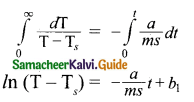Where b1 is the constant of integration. Taking exponential both sides, we get
T = Ts + b2 $$e^{-\frac{a}{m s} t}$$
here b2 = $$e^{b_{1}}$$ = constant.

Question 8.
Explain Wien’s law and why our eyes are sensitive only to visible rays?
Wien’s law states that, ‘the wavelength of maximum intensity of emission of a black body radiation is inversely proportional to the absolute temperature of the black body’.
λm ∝ $$\frac { 1 }{ T }$$
(or)
λm = $$\frac { b }{ T }$$ … (1)
It is implied that if temperature of the body increases, maximal intensity wavelength (λm) shifts towards lower wavelength (higher frequency) of electromagnetic spectrum.

Wien’s law and Vision: Why our eye is sensitive to only visible wavelength (in the range 400 nm to 700nm)?

The Sun is approximately considered as a black body. Since any object above 0 K will emit radiation, Sun also emits radiation. Its surface temperature is about 5700 K. By substituting this value in the equation (1),It is the wavelength at which maximum intensity is 508nm. Since the Sun’s temperature is around 5700K, the spectrum of radiations emitted by Sun lie between 400 nm to 700 nm which is the visible part of the spectrum.

The humans evolved under the Sun by receiving its radiations. The human eye is sensitive only in the visible.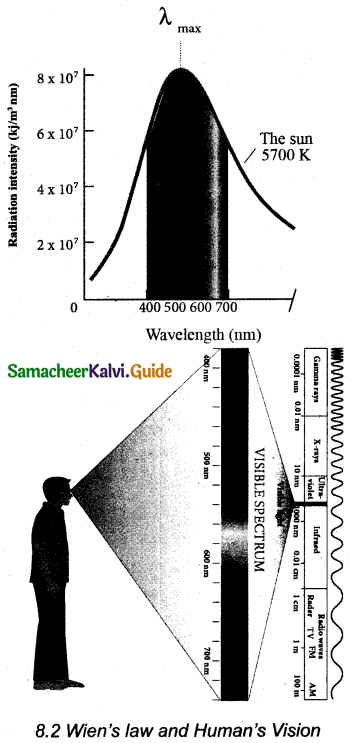Question 9.
Discuss the,
(i) Thermal equilibrium
(ii) Mechanical equilibrium
(iii) Chemical equilibrium
(iv) Thermodynamic equilibrium.
(i) Thermal equilibrium: Two systems are said to be in thermal equilibrium with each other if they are at the same temperature, which will not change with time.

(ii) Mechanical equilibrium: A system is said to be in mechanical equilibrium if no unbalanced force acts on the thermodynamic system or on the surrounding by thermodynamic system.

(iii) Chemical equilibrium: If there is no net chemical reaction between two thermodynamic systems in contact with each other then it is said to be in chemical equilibrium.

(iv) Thermodynamic equilibrium: If two systems are set to be in thermodynamic equilibrium, then the systems are at thermal, mechanical and chemical equilibrium with each other. In a state of thermodynamic equilibrium the macroscopic variables such as pressure, volume and temperature will have fixed values and do not change with time.

Question 10.
Explain Joule’s Experiment of the mechanical equivalent of heat.
Joule showed that mechanical energy can be converted into internal energy and vice versa. In his experiment, two masses were tied with a rope and a paddle wheel as shown in Figure. When these masses fall through a distance h due to gravity, both the masses lose potential energy which is equal to 2mgh. When the masses fall, the paddle wheel turns. Due to the turning of wheel inside water, frictional force comes in between the water and the paddle wheel.

This causes a rise in temperature of the water. This confirms that gravitational potential energy is converted to internal energy of water. The temperature of water increases due to the work done by the masses. In fact, Joule was able to show that the mechanical work has the same effect as giving heat energy. He found that to raise lg of an object by 1°C , 4.186J of energy is required. In earlier days the heat was measured in calorie.
1 cal = 4.186 J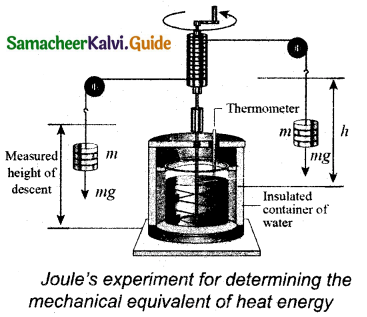This is called Joule’s mechanical equivalent of heat.

Question 11.
Derive the expression for the work done in a volume change in a thermodynamic system.
Let us consider a gas contained in the cylinder fitted with a movable piston. When the gas is expanded quasi-statically by pushing the piston by a small distance dx as shown in Figure. Because the expansion occurs quasi- statically. The pressure, temperature and internal energy will have unique values at every instant.
The small work done by the gas on the piston
dW = F dx … (1)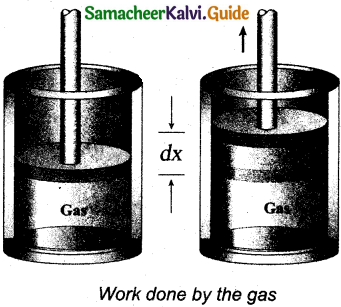The force exerted by the gas on the piston F – PA.
A – area of the piston and
P – pressure exerted by the gas on the piston.
Equation (1) can be rewritten as
dW = PA dx … (2)
But A dx = dV change in volume during this expansion process.
Hence the small work done by the gas during the expansion is given by
dW = dV … (3)
It is noted that is positive since the volume is increased. In general the work done by the gas by increasing the volume from Vi to Vf is given by
W = $$\int_{V_{i}}^{\mathrm{V}_{f}} \mathbf{P} d \mathrm{~V}$$
Suppose if the work is done on the system, then Vi > Vf.
Then, W is negative.Question 12.
Derive Meyer’s relation for an ideal gas.
Let us consider μ mole of an ideal gas in a container with volume V, pressure P and temperature T. When the gas is heated at constant volume the temperature increases by dT. Since no work is done by the gas, the heat that flows into the system will increase only the internal energy. Let the change in internal energy be dU.
If Cv is the molar specific heat capacity at constant volume,.
dU= μCvdT … (1)
When the gas is heated at constant pressure so that the temperature increases by dT. If ‘Q’ is the heat supplied in this process and ‘dV’ the change in volume of the gas.
Q = μCpdT … (2)
If W is the workdone by the gas in this process, then
W = P dV … (3)
But from the first law of thermodynamics,
Q = dU + W … (4)
Substituting equations (1), (2) and (3) in (4), we get,
μCpdT = μCvdT + P dV … (5)
For mole of ideal gas, the equation of state is given by,
PV = μRT
⇒ PdV + VdP = μRdT … (6)
Since the pressure is constant, dP = 0
∴ CpdT = CvdT + RdT
∴ Cp = Cv + R
(or) Cp – Cv = R … (7)
This relation is called Meyer’s relation.

Question 13.
Explain in detail the isothermal process.
It is a process in which both the pressure and volume of a thermodynamic system will change at constant temperature. The ideal gas equation is
PV = μRT
Here, T is constant for this process.
So the equation of state for isothermal process is given by,
PV= constant … (1)
It is implied that if the gas goes from one equilibrium state (P1,V1) to another equilibrium state (P2,V2) the following relation holds for this process
P1,V1 = P2,V2 … (2)
Since PV = constant, P is inversely proportional to V(P ∝ $$\frac { 1 }{ V }$$). This implies that PV graph is a hyperbola. The pressure-volume graph for constant temperature is also called isotherm.
The following figure shows the PV diagram for quasi-static isothermal expansion and quasi-static isothermal compression. For an isothermal process since temperature is constant, the internal energy is also constant. It implies that dU or ∆U – 0.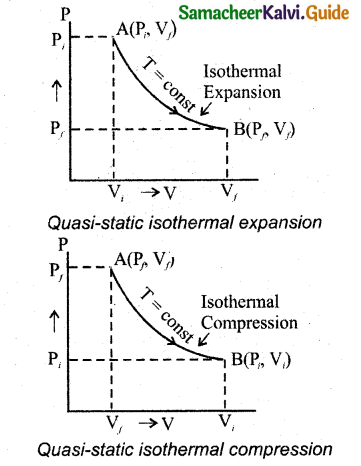For an isothermal process, the first law of thermodynamics can be written as follows,
Q = W … (3)
From equation (3), it implies that the heat supplied to a gas is used to do only external work. It is a common misconception that when there is flow of heat energy to the system, the temperature will increase. For isothermal process it is not true. When the piston of the cylinder is pushed, the isothermal compression takes place. This will increase the internal energy which will flow out of the system through thermal contact.Question 14.
Derive the work done in an isothermal process.
Let us consider an ideal gas which is allowed to expand quasi-statically at constant temperature from initial state (Pi,Vi) to the final state (Pf, Vf. Let us calculate the work done by the gas during this process.
gas, W = $$\int_{V_{i}}^{\mathrm{V}_{f}} \mathrm{P} d \mathrm{~V}$$ … (1)
As the process occurs quasi-statically, at every stage the gas is at equilibrium with the surroundings. Since it is in equilibrium at every stage the ideal gas law is valid.
P = $$\frac { μRT }{ V }$$ … (2)
Substituting equation (2) and (1) we get,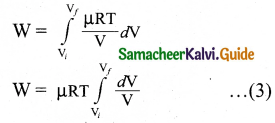In equation (3), μRT is taken out of the integral, since it is constant throughout the isothermal process.
By performing the integration in equation (3), we get
W = μRTln$$\left(\frac{\mathrm{V}_{f}}{\mathrm{~V}_{i}}\right)$$ … (4)
Since we have an isothermal expansion,
$$\frac{\mathrm{V}_{f}}{\mathrm{~V}_{i}}$$ > 1, so $$\left(\frac{\mathrm{V}_{f}}{\mathrm{~V}_{i}}\right)$$ > 0. As a result the work done by the gas during an isothermal expansion is positive.
Equation (4) is true for isothermal compression also. But in an isothermal compression $$\frac{\mathrm{V}_{f}}{\mathrm{~V}_{i}}$$ < 1. So $$\left(\frac{\mathrm{V}_{f}}{\mathrm{~V}_{i}}\right)$$ < 0.
Hence the work done on the gas in an isothermal compression is negative. In the PV diagram the work done during the isothermal expansion is equal to the area under the graph as shown in figure.Similarly for an isothermal compression, the area under the PV graph is equal to the work done on the gas that turns out to be the area with a negative sign.

Question 15.
Explain in detail an adiabatic process.
It is a process in which no heat energy flows into or out of the system (Q = 0). But the gas can expand by spending its internal energy or gas can be compressed through some external work. Hence the pressure, volume and temperature of the system may change in an adiabatic process.

From the first law for an adiabatic process, ∆U = W. This implies that the work is done by the gas at the expense of internal energy or work is done on the system that increases its internal energy. The adiabatic process can be achieved by the following methods

(i) Thermally insulating the system from surroundings. So that no heat energy flows into or out of the system. For instance, when thermally insulated cylinder of gas is compressed (adiabatic compression) or expanded (adiabatic expansion) as shown in the Figure.(ii) If the process occurs so quickly so that there is no time to exchange heat with surroundings even though there is no thermal insulation

The equation (1). Implies that if the gas goes from an equilibrium state (Pi, Vi) to another equilibrium state (Pf,Vf) adiabatically then it satisfies the relation,
PVγ = constant … (1)
The equation (1) Here γ is called adiabatic exponent ( γ = $$\frac{C_{\mathrm{P}}}{C_{\mathrm{V}}}$$) which depends on the nature of the gas.
The equation (1). Implies that if the gas goes from an equilibrium state (Pi,Vi) to another equilibrium state (Pf, Vf) adiabatically then it satisfies the relation,
PiViγ = PfVfγ … (2)The PV diagram for an adiabatic process is also called adiabatic. It is noted that the PV diagram for isothermal and adiabatic processes look similar. But actually the adiabatic curve is steeper than isothermal curse.
Let us rewrite the equation (1) in terms of T and V. From ideal gas equation, the pressure P = $$\frac { μRT }{ V }$$. Substituting this equation in the equation (1), we have
$$\frac { μRT }{ V }$$Vγ = constant
(or) $$\frac { T }{ V }$$Vγ = $$\frac { constant }{ μR }$$
Note here that is another constant. So it can
be written as
TVγ-1 = constant … (3)
From the equation (3) it is known that if the gas goes from an initial equilibrium state (Ti,Vi) to final equilibrium state (Tf, Vf) adiabatically then it satisfies the relation
$$\mathrm{T}_{i} \mathrm{~V}_{i}^{\gamma-1}=\mathrm{T}_{f} \mathrm{~V}_{f}^{\gamma-1}$$ … (4)
The equation of state for adiabatic process can also be written in terms of T arid P as
$$\mathrm{T}^{\gamma} \mathrm{P}^{1-\gamma}$$ = constant

Question 16.
Derive the work done in an adiabatic process.
Let us consider p moles of an ideal gas enclosed in a cylinder having perfectly non conducting walls and base. A frictionless and insulating piston A is fitted in the cylinder as shown in Figure. It’s area of cross-section be A.Let W be the work done when the system goes from the initial state (PiViTi) to the final state (PfVfTf) adiabatically.
W = $$\int_{V_{i}}^{\mathrm{V}_{f}} \mathrm{P} d \mathrm{~V}$$ … (1)
It is assumed that the adiabatic process occurs quasi-statically, at every stage the ideal gas law is valid. Under this condition, the adiabatic equation of state is PVγ = constant (or) P = $$\frac{\text { constant }}{\mathrm{V}^{\gamma}}$$ can be substituted in the equation (1), we get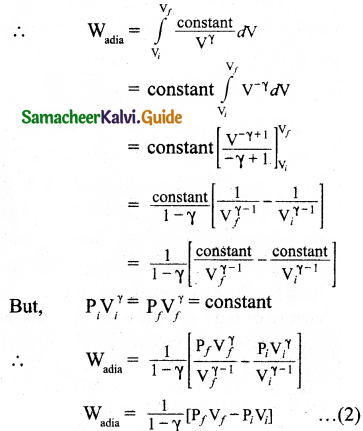From ideal gas law,In adiabatic expansion, work is done by the gas. i.e., Wadia is positive. As Ti > Tf the gas cools during adiabatic expansion.

In adiabatic compression, work is done on the gas. i.e., Wadia is negative. As Ti < Tf hence the temperature of the gas increases during adiabatic compression.Question 17.
Explain the isobaric process and derive the work done in this process.
Isobaric process is a thermodynamic process that occurs at constant pressure. Inspite of the pressure consistency of this process, temperature, volume and internal energy are not constant. From the ideal gas equation, we have
V = ($$\frac { μR }{ P }$$)T … (1)
Here, $$\frac { μR }{ P }$$ = constant
In an isobaric process the temperature is directly proportional to volume.
V ∝ T(Isobaric process) … (2)
It is implied that for a isobaric process, the V-T graph is a straight line passing through the origin.
Work done by the gas
W= $$\int_{V_{i}}^{\mathrm{V}_{f}} \mathrm{P} d \mathrm{~V}$$
In an isobaric process, the pressure is constant, So P comes out of the integral,∆V – change in the volume. If AV is negative, W is also negative. It is implied that the work is done on the gas. If ∆V is positive, W is also positive, implying that work is done by the gas. From the ideal gas equation.
equation (4) can be rewritten as
PV = μRT and V = $$\frac { μRT }{ P }$$
Substituting this in equation (4) we get,
W = μRTf(1 – $$\frac{\mathrm{T}_{i}}{\mathrm{~T}_{f}}$$) … (5)
In the PV diagram, area under the isobaric curve is equal to the work done in isobaric process. The shaded area in the following Figure is equal to the work done by the gas.The first law of thermodynamics for isobaric process is given by,
∆U = Q – P∆V

Question 18.
Explain in detail the isochoric process.
Isochoric process is a thermodynamic process in which the volume of the system is kept constant. But pressure, temperature and internal energy continue to be variables. The pressure-volume graph for an isochoric process is a vertical line parallel to pressure axis as shown in Figure.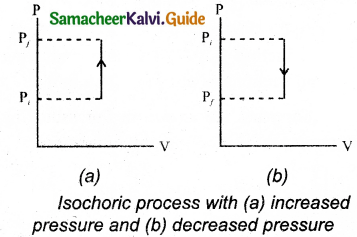The equation of state for an isochoric process is given by,
P = $$\frac { μR }{ V }$$ … (1)
Here $$\frac { μR }{ V }$$ = constant
∴ P ∝ T
It is inferred that the pressure is directly proportional to temperature. This implies that the P-T graph for an isochoric process is a straight line passing through origin. If a gas goes from state (Pi, Ti) to (Pf, Tf) at constant volume, then the system satisfies the following equation,
$$\frac{P_{i}}{T_{i}}=\frac{P_{f}}{T_{f}}$$
For an isochoric processes, AV=0 and W-0. Then the first law of thermodynamics becomes
∆U = Q … (3)
It is implied that the heat supplied is used to increase only the internal energy. As a result the temperature increases and pressure also increases.

Question 19.
What are the limitations of the first law of thermodynamics?
The first law of thermodynamics explains well the inter convertibility of heat and work. But is not indicated the direction of change.
Example:
(i) When a hot object is in contact with a cold object, heat always flows from the hot object to cold object but not in the reverse direction. According to first law of thermodynamics, it is possible for the energy to flow from hot object to cold object and viceversa. But in nature the direction of heat flow is always from higher temperature to lower temperature.

(ii) When brakes are applied, a car stops due to friction and the work done against friction is converted into heat. But this heat is not reconverted into the kinetic energy of the car. Hence the first law is not sufficient to explain many of natural phenomena.Question 20.
Explain the heat engine and obtain its efficiency.
Heat engine is a device which takes heat as input and converts this heat into work by undergoing a cyclic process.
A heat engine has three parts:
(i) Hot reservoir
(ii) Working substance
(iii) Cold reservoir
A Schematic diagram for heat engine is given below in the figure.• Hot reservoir (or) Source: It supplies heat to the engine. Which is always maintained at a high temperature TH.
• Working substance: It is a substance like gas or water, that converts the heat supplied into work.
• Cold reservoir (or) Sink: The heat engine ejects some amount of heat (QL) into cold reservoir after it doing work. It is always maintained at a low temperature TL.

The heat engine works in a cyclic process. After a cyclic process it returns to the same state. Since the heat engine returns to the same state after it ejects heat, the change in the internal energy of the heat engine is zero.

The efficiency of the heat engine is defined as the ratio of the work done (output) to the heat absorbed (input) in one cyclic process. Let the working substance absorb heat QH units from the source and reject QL units to the sink after doing work W units.
We can write,
Input heat = Work done + ejected heat
QH = W + QL
W = QH – QL
Then the efficiency of heat engine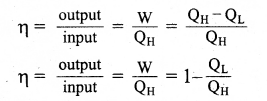Question 21.
Explain in detail Carnot heat engine.
A reversible heat engine operating in a cycle between two temperatures in a particular way is called a Carnot Engine. The carnot engine consists of four parts that are given below.

1. Source: It is the source of heat maintained at constant high temperature TH. Without changing its temperature any amount of heat can be extracted from it.
2. Sink: It is a cold body maintained at a constant low temperature TL. It can absorb any amount of heat.
3. Insulating stand: It is made of perfectly non-conducting material. Heat is not conducted through this stand.
4. Working substance: It is an ideal gas enclosed in a cylinder with perfectly non-conducting walls and perfectly conducting bottom. A non-conducting and frictionless piston is fitted in it.

The four parts are shown in the following Figure.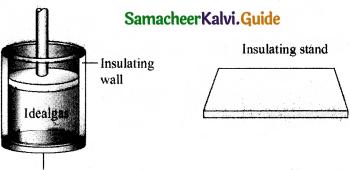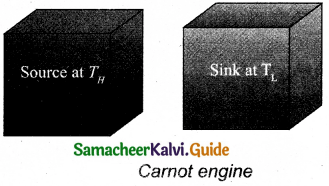Carnot’s cycle: In camot’s cycle, the working substance is subjected to four successive reversible processes.
Let the initial pressure, volume of the working substance be P1, V1.
When the cylinder is placed on the source, the heat (QH) flows from source to the working substance (ideal gas) through the bottom of the cylinder.
The input heat increases the volume of the gas.So the piston is allowed to move out very’ slowly.
W1 is the work done by the gas it expands from volume V1 to volume V2 with a decrease of pressure from P1 to P2.
Then the work done by the gas (working substance) is given by
∴ QH = QA→B $$\int_{V_{1}}^{\mathrm{V}_{2}} \mathrm{P} d \mathrm{~V}$$
When the cylinder is placed on the insulating stand and the piston is allowed to move out, the gas expands adiabatically from volume V2 to volume V3 the pressure falls from P2 to P3. The temperature falls to TLK.
The work done by the gas in an adiabatic expansion is given by,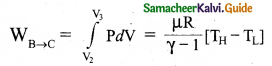Area under the curve BC.
Isothermal compression from (P3, V3, TL) to (P4, V4, TL).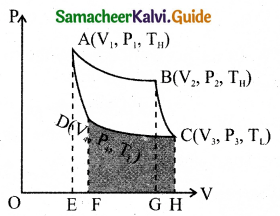Let the cylinder is placed on the sink and the gas is isothermally compressed until the pressure and volume become P4 and V4 respectively.Let the cylinder is placed on the insulating stand again and the gas is compressed adiabatically till it attains the initial pressure P1, volume V2 and temperature TH, Which is shown by the curve DA in the P – V diagram.= – Area under the curve DA
Let ‘W’ be the net work done by the working substance in one cycle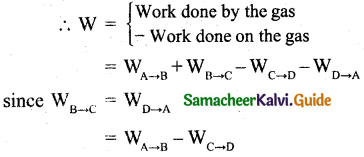The net work done by the Carnot engine in one cycle is given by,
W = WA→B – WC→D
Net work done by the working substance in one cycle is equal to the area (enclosed by ABCD) of the P-V diagram.Question 22.
Derive the expression for Carnot engine efficiency.
Efficiency is defined as the ratio of work done by the working substance in one cycle to the amount of heat extracted from the source.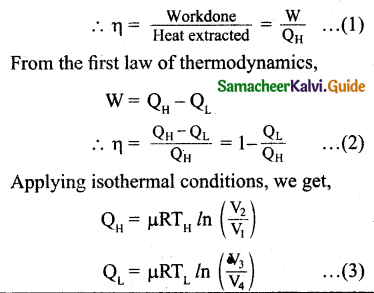Let us omit the negative sign. Since we are interested in only the amount of heat (QL) ejected into the sink, we have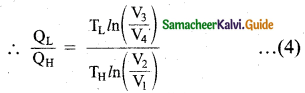By applying adiabatic conditions, we get,
TH V2γ-1 = TL V3γ-1
TH V1γ-1 = TL V4γ-1
By dividing the above two equations, we get
$$\left(\frac{\mathrm{V}_{2}}{\mathrm{~V}_{1}}\right)$$γ-1 = $$\left(\frac{\mathrm{V}_{3}}{\mathrm{~V}_{4}}\right)$$γ-1
Which implies that
$$\frac{\mathrm{V}_{2}}{\mathrm{~V}_{1}}=\frac{\mathrm{V}_{3}}{\mathrm{~V}_{4}}$$ … (5)
Substituting equation (5) and (4), we get
$$\frac{\mathrm{Q}_{\mathrm{L}}}{\mathrm{Q}_{\mathrm{H}}}=\frac{\mathrm{T}_{\mathrm{L}}}{\mathrm{T}_{\mathrm{H}}}$$
∴ The efficiency
η = 1 – $$\frac{\mathrm{T}_{\mathrm{L}}}{\mathrm{T}_{\mathrm{H}}}$$

Question 23.
Explain the second law of thermodynamics in terms of entropy.
$$\frac{\mathrm{Q}_{\mathrm{L}}}{\mathrm{Q}_{\mathrm{H}}}$$ = $$\frac{\mathrm{T}_{\mathrm{L}}}{\mathrm{T}_{\mathrm{H}}}$$ … (1)
According to efficiency of Carnot’s engine equation, quantity $$\frac{\mathrm{Q}_{\mathrm{H}}}{\mathrm{T}_{\mathrm{H}}}$$ = $$\frac{\mathrm{Q}_{\mathrm{L}}}{\mathrm{T}_{\mathrm{L}}}$$.
The quantity $$\frac { Q }{ T }$$ is called entropy. It is a very important thermodynamic property of a System, which is also a state variable.
$$\frac{\mathrm{Q}_{\mathrm{H}}}{\mathrm{T}_{\mathrm{H}}}$$ is the entropy received by the Carnot engine from hot reservoir and $$\frac{\mathrm{Q}_{\mathrm{L}}}{\mathrm{T}_{\mathrm{L}}}$$ is entropy given out by the Carnot engineLto the cold reservoir. For reversible engines (Carnot Engine) both entropies should be same, so that the change in entropy of the Carnot engine in one cycle is zero. It is proved in equation (1).
But for all practical engines like diesel and petrol engines which are not reversible engines, they satisfy the relation $$\frac{\mathrm{Q}_{\mathrm{L}}}{\mathrm{T}_{\mathrm{L}}}$$ > $$\frac{\mathrm{Q}_{\mathrm{H}}}{\mathrm{T}_{\mathrm{H}}}$$.
In fact the second law of thermodynamics can be reformulated as follows “For all the processes that occur in nature(irreversible process), the entropy always increases. For reversible process entropy will not change”. Entropy determines the direction in which natural process must occur.

Question 24.
Explain in detail the working of a refrigerator.
A refrigerator is a Carnot’s engine working in the reverse order which is shown in the figure.8.17 Schematic diagram of a refrigerator The working substance (gas) absorbs a quantity of heat QL from the cold body (sink) at a lower temperature TL. A certain amount of work W is done on the working substance by the compressor and a quantity of heat QH is rejected to the hot body (source) i.e., the atmosphere at TH. When we stand beneath of the refrigerator, we can feel warmth air.
From the first law of thermodynamics, we have QL + W = QH
Hence the cold reservoir (refrigerator) further cools down and the surroundings (kitchen or atmosphere) gets hotter.IV. Numerical problems:

Question 1.
Calculate the number of moles of air is in the inflated balloon at room temperature as shown in the figure.The radius of the balloon is 10 cm, and pressure inside the balloon is 180 kPa.
The pressure inside the balloon P = 1.8 x 105 P
Room temperature
T = 273 + 30 = 303K.
Let the radius of the balloon be R = 10 x 10-2m.
Volume of the balloon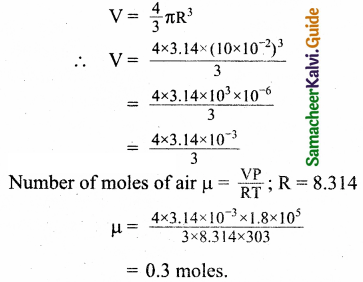Question 2.
In the planet Mars, the average temperature is around – 53°C and atmospheric pressure is 0.9 kPa. Calculate the number of moles of
the molecules in unit volume in the planet Mars? Is this greater than that in earth?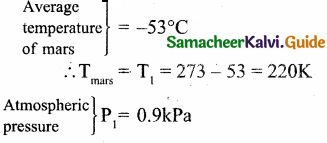= 0.9 x 10³Pa
PV = NkT
Since volume is unity V = 1
Equation (1) becomes
P = NkT
Where k is Boltzmann constantQuestion 3.
An insulated container of gas has two chambers separated by an insulating partition. One of the chambers has volume V1 and contains ideal gas at pressure P1 and temperature T1. The other chamber has volume V1 and contains ideal gas at pressure P2 and temperature T2. If the partition is removed without doing any work on the gases, calculate the final equilibrium temperature of the container.
In first chamber let the pressure be P1
In second chamber let the pressure be P2
In first chamber let the volume be V1
In second chamber let the volume be V2
In first chamber let the temperature be T1
In second chamber let the temperature be T2
According to conservation of energy,Question 4.
The temperature of a uniform rod of length L having a coefficient of linear expansion αL is changed by ∆T. Calculate the new moment of inertia of the uniform rod about axis passing through its centre and perpendicular to an axis of the rod.
Moment of inertia of uniform rod of mass M, and length L about axis passing through its centre and perpendicular to an axis of rod is given by
I = $$\frac{\mathrm{ML}^{2}}{12}$$
The increase in length of the rod
∆l = LαL∆T
αL – Coefficient of linear expansion.
∆T – change in temperature
After the rod is heated,
Moment of inertia,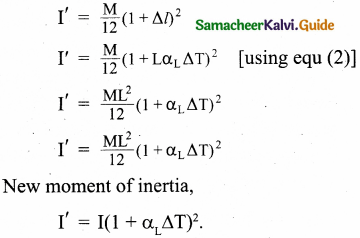Question 5.
Draw the TP diagram (P-x axis, T-y axis), VT(T-x axis, V-y axis) diagram for
(a) Isochoric process
(b) Isothermal process
(c) isobaric process.
(a) For isochoric process:
V = V0 = constant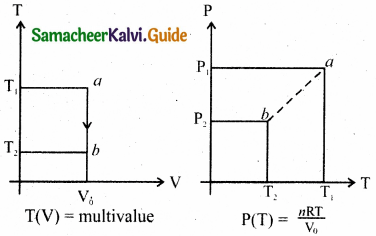The points a and b are represented as a = (P1V0T0) b = (P2, V0, T2)

(b) For isothermal process:
T = T0 = constant
PV = nRT0P(T) = multivalue
P(T) = $$\frac{n \mathrm{RT}_{0}}{\mathrm{~V}}$$
The points a and b are represented as
a = (P1V1,T) b = (P2V0T0)

(c) For isobaric process:
P = P0 = constant
T(V) = $$\frac{\mathrm{P}_{0} \mathrm{~V}}{n^{2}}$$
P0V = nRT
P(T) = P0Question 6.
A man starts bicycling in the morning at a temperature around 25°C, he checked the pressure of tire which is equal to be 500 kPa. Afternoon he found that the absolute pressure in the tyre is increased to 520 kPa. By assuming the expansion of tyre is negligible, what is the temperature of tyre at afternoon?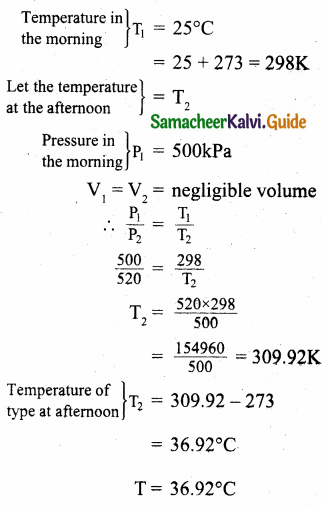Question 7.
The temperature of a normal human body is 98.6°F. During high fever if the temperature increases to 104°F, what is the change in peak wavelength that emitted by our body? (Assume human body is a black body)
Normal temperature of human body = 98.6°F
Conversion of F° to C°
C = $$\frac { (F-32) }{ 1.8 }$$
= $$\frac { (98.6-32 }{ 1.8 }$$
= $$\frac { 66.6 }{ 1.8 }$$
= 37°C
Conversion of C° to Kelvin
T = 37 + 273 = 310
Peak wavelength at 98.6°F is
λm = $$\frac { b }{ T }$$
= $$\frac{2.898 \times 10^{-3}}{310}$$
= 0.009348 x 10-3 m
= 9348 x 10-9
= 9348 nm
Final temperature of the human body = 104°F
Conversion of F° to C°
C = $$\frac { F-32 }{ 1.8 }$$
= $$\frac { 104-32 }{ 1.8 }$$
= $$\frac { 72) }{ 1.8 }$$
Conversion of 40° to Kelvin
T = 40+ 273 = 313 K
Peak wavelength at 104°F is
λm = $$\frac { b }{ T }$$
= $$\frac{2.898 \times 10^{3}}{313}$$
= 0.009258 x 10-3 m
= 9258 x 10-9 = 9258 nm
λmax = 9348 nm at 98.6°F
λmax = 9258 nm at 104°FQuestion 8.
In an adiabatic expansion of the air, the volume is increased by 4%, what is percentage change in pressure? (For air γ = 1.4)
Percentage of increased volume = 4%
$$\frac { ∆V }{ V }$$ x 100 = 4%
γ = 1.4
PVγ = ConstantQuestion 9.
In a petrol engine, (internal combustion engine) air at atmospheric pressure and temperature of 20°C is compressed in the cylinder by the piston to 1/8 of its original volume. Calculate the temperature of the compressed air. (For air γ = 1.4)
P1 = 1 atmospheric pressure, V1 = V, V2 = $$\frac { V }{ 8 }$$
T1 = 20 + 273 – 293K, T2 – ?, γ = 1.4.∴ The temperature of the compressed air = 400°C

Question 10.
Consider the following cyclic process consist of isotherm, isochoric and isobar which is given in the figure.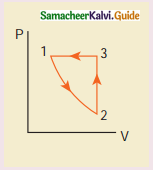Draw the same cyclic process qualitatively in the V-T diagram where T is taken along x direction and V is taken along y-direction. Analyze the nature of heat exchange in each process.

Process 1 to 2: increase in volume. So heat must be added.

Process 2 to 3: Volume remains constant. Increase in temperature. The given heat is used to increase the internal energy.

Process 3 to 1: Pressure remains constant. Volume and Temperature are reduced. Heat flows out of the system. It is an isobaric compression where the work is done on the system.Explanation: In the graph, during the process (1 to 2), the gas undergoes isothermal expansion. It receives certain amount of heat from the surroundings. It uses this heat in doing the work. Hence internal energy of the gas remains unchanged.

During the process represented by (2 to 3) the gas is heated at constant volume. Since no work is done and volume does not change, the process is isochoric process.

Since heat is transferred to the gas from the surroundings, the internal energy of the gas is increased. During the process represented by (3 to 1) the gas is compressed isobarically. Work is done on the gas. Since temperature drops internal energy is reached. Hence the gas gives up heat to the surroundings.

Question 11.
An ideal gas is taken in a cyclic process as shown in the figure. Calculate
(a) work done by the gas.
(b) work done on the gas.
(c) Net work done in the process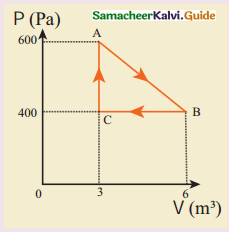(a) Let the work done by the gas along AB be W. From the fig pressure,
P = 600 N/m²
From the fig change in volume,
∆V = 3
∴Work done,
W = PAV
= 600 x 3 = 1800J
= 1.8 kJ

(b) Isobaric compression take place and work is done on the gas along BC.
W = – PAV
∆V = (6 – 3) = 3
P = +400N/m²
∴ W= – 400 x 3
= – 1200
= – 1.2 kJ

(c)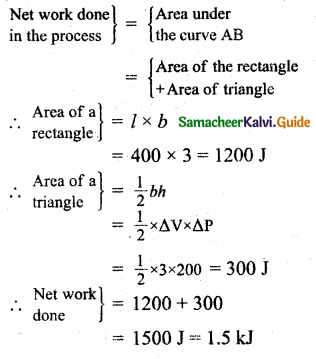Question 12.
For a given ideal gas 6 x 105 J heat energy is supplied and the volume of gas is increased from 4 m³ to 6 m² at atmospheric pressure. Calculate
(a) the work done by the gas
(b) change in internal energy of the gas
(c) graph this process in PV and TV diagram.
Heat energy supplied to the gas
Q = 6 x 105J

(b) Change in internal energy
∆U = ∆Q – AW
= ∆Q – P∆V
= 6 x 105 – 2.026 x 105
= 3,974 x 103
∆U = 3.974 kJ

(c)Question 13.
Suppose a person wants to increase the efficiency of the reversible heat engine that is operating between 100°C and 300°C. He had two ways to increase the efficiency.
(a) By decreasing the cold reservoir temperature from 100°C to 50°C and keeping the hot reservoir temperature constant
(b) By increasing the temperature of the hot reservoir from 300°C tc 350°C by keeping the cold reservoir temperature constant. Which is the suitable method?
Temperature of cold reservoir
T1 = 100°C
= 100 + 273 – 373 K
Temperature of hot reservoir
TH = 300 + 273 = 573 K
η = 1 – $$\frac{\mathrm{T}_{\mathrm{L}}}{\mathrm{T}_{\mathrm{H}}}$$
η = 1 – $$\frac { 373 }{ 573 }$$
η = 1 – $$\frac { 573 – 373 }{ 573 }$$
= $$\frac { 200 }{ 753 }$$
= 0.349 x 100
Initial efficiency = 34.9%

(a) By decreasing the temperature of the cold reservoir from 100°C to 50PC.(b) By increasing the temperature of the hot reservoir from 300°C to 350°C,
T1 = 350 + 273 = 623K
T1 = 100 + 273 =373K
∴ Efficiency,
η = 1 – $$\frac{\mathrm{T}_{\mathrm{2}}}{\mathrm{T}_{\mathrm{1}}}$$
η = 1 – $$\frac { 373 }{ 623 }$$
η = $$\frac { 623 – 373 }{ 623 }$$
η = $$\frac { 250 }{ 623 }$$
η = 0.4012
η = 0.4012 x 100 = 40.12%
∴ Method (a) is more efficient than method (b).Question 14.
A Carnot engine whose efficiency is 45% takes heat from a source maintained atatemperature of 327°C. To have an engine of efficiency 60% what must be the intake temperature for the same exhaust (sink) temperature?
Temperature of a source,
T1 = 327°C = 327 + 273 = 600 k
η = $$\frac { 45 }{ 100 }$$ = 0.45
Efficiency η = 1 – $$\frac{\mathrm{T}_{\mathrm{2}}}{\mathrm{T}_{\mathrm{1}}}$$
∴ 0.45 = 1 – $$\frac{\mathrm{T}_{2}}{600}$$
$$\frac{\mathrm{T}_{2}}{600}$$ = 1 – 0.45 = 0.55
∴ T2 = 0.55 x 600 = 330 K
η = 60% = $$\frac { 60 }{ 100 }$$ = 0.6
0.6 = 1 – $$\frac{\mathrm{T}_{\mathrm{2}}}{\mathrm{T}_{\mathrm{1}}}$$ = 1 – $$\frac{330}{\mathrm{~T}_{1}}$$
$$\frac{330}{\mathrm{~T}_{1}}$$ = 1 – 0.6 = 0.4
In take temperature
T1 = $$\frac { 330 }{ 0.4 }$$ = 825K
= 825 – 273
= 552°C
The intake temperature = 552°C

Question 15.
An ideal refrigerator keeps its content at 0°C while the room temperature is 27°C. Calculate its coefficient of performance.